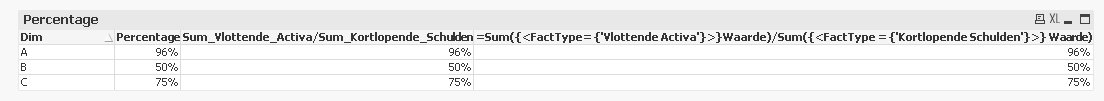# QlikView App Development

Discussion Board for collaboration related to QlikView App Development.

Highlighted
Contributor

## Precalculate expressions in script

Hi guys,

I have a quick question and I cannot find a definitive answer.

is it possible to calculate expressions that are used in charts in the load script? as within a preceding load?

I have this expression:

=sum({<FactType= {'Vlottende Activa'}>}Waarde)/SUM({<FactType = {'Kortlopende Schulden'}>} Waarde)

can I in some way precalculate this and put this into a field? and if this is possible is it even useful to do this? Could this potentially speed up my app? or is it going to be slower if I do this for every graph. The problem right now is that my app needs 1.5 seconds to change when I change a filter. it has about 3.5 mln facts with each set it own facttype, the fact table it self only has 16 fields. It shouldn't be this slow, it is even worse when it is on a server.

Jasper

Tags (3)
1 Solution

Accepted SolutionsMVP

## Re: Precalculate expressions in script

It all depends on what your dimensions are and you can pre-calculate the Sums or even the division. Here is a small sample for you where I am assuming that you want to aggregate this on the Dim dimension

Table:

Dim, FactType, Waarde

A, Vlottende Activa, 10

A, Kortlopende Schulden, 15

A, Vlottende Activa, 20

A, Kortlopende Schulden, 10

A, Vlottende Activa, 23

A, Kortlopende Schulden, 30

B, Vlottende Activa, 30

B, Vlottende Activa, 20

B, Kortlopende Schulden, 60

B, Kortlopende Schulden, 40

C, Vlottende Activa, 30

C, Kortlopende Schulden, 40

];

Left Join (Table)

Sum(If(FactType = 'Vlottende Activa', Waarde)) as Sum_Vlottende_Activa,

Sum(If(FactType = 'Kortlopende Schulden', Waarde)) as Sum_Kortlopende_Schulden,

Sum(If(FactType = 'Vlottende Activa', Waarde))/Sum(If(FactType = 'Kortlopende Schulden', Waarde)) as Percentage

Resident Table

Group By Dim;

Now for comparison, I will show you how this will look in three different scenariosDimension

Dim

Expressions

1) Percentage

2) Sum_Vlottende_Activa/Sum_Kortlopende_Schulden

3) =Sum({<FactType= {'Vlottende Activa'}>}Waarde)/Sum({<FactType = {'Kortlopende Schulden'}>} Waarde)

But since now we have done this for Dim dimension, we probably won't be able to use this for any other dimension. Lets say we want to use them in a dimension less text box and you will see that you won't be able to replicate the result of the third expression with the other two expressions. Unless you create another set of aggregation, you won't be able to use it in text box object.

So, in short if you know what the dimensions are going to be, then go ahead with this. Else, this could become very challenging very quickly specially the percentage. Sums show still be good.

HTH

Best,

Sunny

5 RepliesMVP

## Re: Precalculate expressions in script

It is possible to do this, but there might be multiple steps involved using Resident Load with Group By statements and where or if clauses.

Valued Contributor III

## Re: Precalculate expressions in script

Hey there,

You can make this like Sunny T have told you, but you do this using flags in preceding loads like this:

Table:

(sum(Waarde) * Flag1) /  (sum(Waarde) * Flag2) as YourSetExpression;

if(FactType = 'Vlottende Activa', 1, 0) as Flag1,

if(FactType = 'Kortlopende Schulden', 1, 0) as Flag2;

Something so simple as this can solve you many problems in performance. Try to bring to script as maximum complexity as you can, so the rendering in charts decrease in time and you'll get the best performance in your dashboard.

Best regards,

Data Architect MB

Contributor

## Re: Precalculate expressions in script

Hi Miguel,

I tried your approach first, how ever when I try to reload the script it gives me an invalid expression error. Though it seems like everything is set up correctly.

```LOAD
*,
(sum(Waarde)*[Vlottende activa])/(sum(Waarde)*[Kortlopende schulden]) as [Liquiditeit];
*,
If(FactType = 'Vlottende Activa', 1,0) as [Vlottende activa],
If(FactType = 'Kortlopende Schulden', 1,0) as [Kortlopende schulden]
```

am I missing something here?

Jasper

Contributor

## Re: Precalculate expressions in script

Hi sunny T,

Thank you for your reply, is there any discussion where an example is given by any chance? or do you have a quick syntax example?

JasperMVP

## Re: Precalculate expressions in script

It all depends on what your dimensions are and you can pre-calculate the Sums or even the division. Here is a small sample for you where I am assuming that you want to aggregate this on the Dim dimension

Table:

Dim, FactType, Waarde

A, Vlottende Activa, 10

A, Kortlopende Schulden, 15

A, Vlottende Activa, 20

A, Kortlopende Schulden, 10

A, Vlottende Activa, 23

A, Kortlopende Schulden, 30

B, Vlottende Activa, 30

B, Vlottende Activa, 20

B, Kortlopende Schulden, 60

B, Kortlopende Schulden, 40

C, Vlottende Activa, 30

C, Kortlopende Schulden, 40

];

Left Join (Table)

Sum(If(FactType = 'Vlottende Activa', Waarde)) as Sum_Vlottende_Activa,

Sum(If(FactType = 'Kortlopende Schulden', Waarde)) as Sum_Kortlopende_Schulden,

Sum(If(FactType = 'Vlottende Activa', Waarde))/Sum(If(FactType = 'Kortlopende Schulden', Waarde)) as Percentage

Resident Table

Group By Dim;

Now for comparison, I will show you how this will look in three different scenariosDimension

Dim

Expressions

1) Percentage

2) Sum_Vlottende_Activa/Sum_Kortlopende_Schulden

3) =Sum({<FactType= {'Vlottende Activa'}>}Waarde)/Sum({<FactType = {'Kortlopende Schulden'}>} Waarde)

But since now we have done this for Dim dimension, we probably won't be able to use this for any other dimension. Lets say we want to use them in a dimension less text box and you will see that you won't be able to replicate the result of the third expression with the other two expressions. Unless you create another set of aggregation, you won't be able to use it in text box object.

So, in short if you know what the dimensions are going to be, then go ahead with this. Else, this could become very challenging very quickly specially the percentage. Sums show still be good.

HTH

Best,

Sunny